# Stars and Bars: Counting Ways to Distribute Items

We have been looking at ways to count possibilities (combinatorics), including a couple ways to model a problem using blanks to fill in. Today, we’ll consider a special model called Stars and Bars, which can be particularly useful in certain problems, and yields a couple useful formulas. (I only remember the method, not the formulas.)

## Balls in urns

We’ll start with a simple example from 2001 that introduces the method:

Placing Balls in Urns

The number of different ways we can place b indistinguishable balls into u distinguishable urns is:

C(b+u-1,b) = C(b+u-1,u-1)

where the notation C(n,r) means the number of ways to choose r things from n things, i.e., the usual binomial coefficient.

“Balls in urns” are a classic way to illustrate problems of this type; today, I rarely see the word “urn” outside of combinatorics, and more often use words like “boxes” or “bags” or “bins”. Picture, say, 3 baskets in a row, and 5 balls to be put in them. The balls are all alike (“indistinguishable”), so we don’t know or care which is in which basket; but we do care how many balls are in basket 1, how many in basket 2, and so on.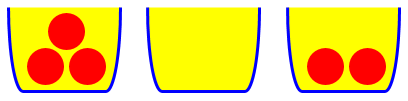We can’t use the most basic approach of counting how many ways there are to place the first ball, and so on, because there is no “first ball” as far as the result is concerned. Nor can we count how many ways there are to fill the first basket, then the next, because the possibilities for one depend on what went before. We need a different model.

Doctor Rob presented the technique:

Number the urns from 1 to u. Line up the indistinguishable balls in a row. Split this row up into u sections by inserting u-1 separators.

All balls to the left of the jth separator and to the right of the (j-1)st separator (counting from, say, the left end) go into the jth urn. You now have a row of b+u-1 objects of which b are balls and u-1 are separators. There are C(b+u-1,b) ways to choose the locations for the b balls among the b+u-1 objects.

Example: 4 balls (o), 3 urns, 2 separators (|):

o o o o | |   (4,0,0)
o o o | o |   (3,1,0)
o o | o o |   (2,2,0)
o | o o o |   (1,3,0)
| o o o o |   (0,4,0)
o o o | | o   (3,0,1)
o o | o | o   (2,1,1)
o | o o | o   (1,2,1)
| o o o | o   (0,3,1)
o o | | o o   (2,0,2)
o | o | o o   (1,1,2)
| o o | o o   (0,2,2)
o | | o o o   (1,0,3)
| o | o o o   (0,1,3)
| | o o o o   (0,0,4)

15 ways, C(6,4) = C(6,2) = 15.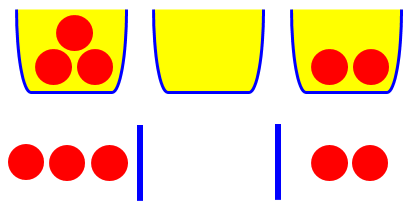My picture above represents the case (3, 0, 2), or “o o o |  | o o”.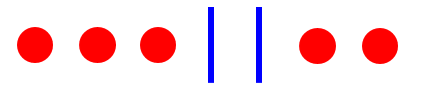Changing our perspective from three urns to 7 symbols, we have b=5, u=3, u-1=2, so we are arranging 7 symbols, which can be thought of as choosing 2 of 7 places to put the separators, with balls in the other places. (Notice how the balls and separators have turned into mere items to be placed in blanks, connecting us back to the most basic model.)

It is common to replace the balls with “stars”, and to call the separators “bars”, yielding the popular name of the technique. We have 5 stars, and 2 bars in our example: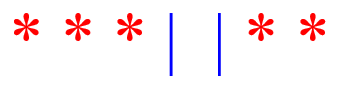I myself have occasionally used “o” and “|”, calling them “sticks and stones”. Or I might call them “balls and walls”. It’s all the same idea.

The formula, using the usual typographic notation, is either $$\displaystyle{{b+u-1}\choose{u-1}}$$, where we choose places for the $$u-1$$ bars, or $$\displaystyle{{b+u-1}\choose{b}}$$, where we choose places for the $$b$$ stars. Clearly, these give the same result, which can also be shown algebraically.

(By the way, it can be instructive to look at the orderly pattern Doctor Rob used to list these possibilities.)

For another introductory explanation, see

Rock, Paper, Scissors

## Boxes of donuts

Now let’s look at a problem in which the technique is a little more abstract:

How Many Different Boxes of Donuts Can Be Made?

A donut shop has exactly 5 different types of donuts. How many
different boxes of a dozen donuts can be made?

Now, I can sit here for ages and write out all of the combos, but I want a formula to help me with this problem. In beginning to solve this problem, I began to think of some easier ways to put the donuts together. I came up with the first five boxes being each of the same kind of donut, and then putting one of each with eleven of the others donuts for ten more boxes. Then two of one type of donut, with only ten of another, making that ten more boxes -- and I know I can keep going with combos of 3 and 9, 4 and 8. However, I would like to find an easier and more efficient way. Thank You!

The numbers here are too large to hope to list the possibilities. Doctor Sam answered this, using stars and bars; he swapped the roles of stars and bars (using the bars as tally marks and stars as separators), which I will change for the sake of consistency here:

Think about taking an order for a dozen donuts from your friends. You might make marks on a sheet of paper like this:

Donut    A   |   B  |   C   |  D  |  E
---------------------------------------
**  |   *  | ****  | *** | **

meaning 2 type As, 1 type B, 4 type C's, 3 type D's and 2 type E's.

Some other possibilities are:

Donut    A   |   B  |   C   |  D  |  E
---------------------------------------
**** |  **  |  ***  |     | ***    (4, 2, 3, 0, 3)
******| **** |       |     | **     (6, 4, 0, 0, 2)

Every possible order can be listed simply by placing 12 marks inside these five columns.

Do you notice something different here? You might have expected the boxes to play the role of “urns”, but they don’t. There is only one box! Instead, our 5 “urns” separated by the 4 bars represent the types of donuts! And the stars are donuts, but they are not placed in boxes but assigned to categories. Why? Because in stars and bars, the stars must be indistinguishable, while the bars separate distinguishable containers. In this problem, the locations don’t matter, but the types of donuts are distinct, so they must be the “containers”.

I like Doctor Sam’s way of introducing the idea here, using as his model not the donuts in a box, but tallies on an order form. (It is because tally marks are typically vertical lines, that he reversed the meaning of the symbols.) Such a concrete model is a great way to make the abstract manageable.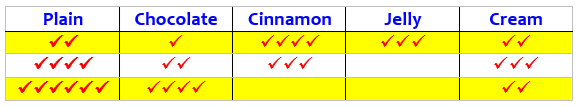He continues:

You have been working out the distributions one-at-a-time. There is an easier way. It depends upon you suddenly seeing the above picture as a list of 16 symbols: twelve * and four |.

ANY list of these 16 symbols can be interpreted as a donut order. For example,

**||*******|***|   means 2 A's, 0 B's, 7 C's, 3 D's, 0 E's

Once you have this idea, the problem is easy, because we have changed it from "number of kinds of donut boxes" to "number of ways of placing 12 *'s and 4 |'s in a row". If you have sixteen places then you can choose a place for the four |'s in C(16,4) ways. Once the |'s are in place, fill in the remaining spaces with *'s. So the answer is 16C4 = 1820.

This method is called "stars and bars."

## Distributing apples: At least one to each

The next problem has a little twist.

Distributing Apples

A number of my friends and I have already wrestled with this one, and have had no success:

There are 8 identical apples, and they are to be given out to 4 children. How many different ways of distributing the apples are there if each child must receive at least one apple?

What we have discussed so far allowed for the possibility that some urns would be empty. What if we disallow that?

Clearly the (indistinguishable) apples will be represented by stars, and the (presumably distinguishable) children are the containers. Doctor Anthony took this first:

Place the 8 apples in a row with gaps between then.  See diagram below.

*   *  |  *  |  *   *    *    *  |  *

To divide the apples into 4 groups we must choose 3 separators '|' to place in the gaps. There are 7 gaps and we must choose 3 from amongst the 7.  This can be done in

C(7,3) = 35  ways

This looks like the same idea, but something is different. Doctor Mitteldorf saw that further explanation would be useful:

Doctor Anthony has shown you an elegant way to do the problem abstractly. The advantage of this approach is that you can see how to extend it to other, similar problems: for example, n identical apples to be distributed among m children.

The trick, in case Doctor Anthony was too concise in his explanation, is this: Imagine the n apples laid out on a grocery checkout counter with n-1 gaps between them. First, put the m children in a particular order in front of the checkout. Then you can put m-1 dividers in to separate which child gets which apples. Since there are n-1 gaps, with m-1 dividers to be inserted, the answer is the same as a combinatorial problem: How many ways can you choose m-1 things out of a list of n-1 in all? This is the number from Pascal's Triangle, often denoted by C(n-1,m-1), or "n-1 choose m-1."

Notice that putting two dividers into one slot would correspond to a child getting no apples, so we don't want to allow that in this case. For the same reason, you can't put a divider at the extreme left end or the extreme right end.

We have the same representation as before, but with the new requirement that no child can be empty-handed, we must require that no two bars can be adjacent. They must be separated by stars. So rather than just freely place bars anywhere, we now think of gaps between stars, and place only one bar (if any) in each gap. For 8 stars and 4 urns (3 bars), we can put bars in any of the 7 spaces between stars (not on the outside, because that would leave an empty urn):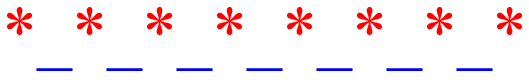One such choice is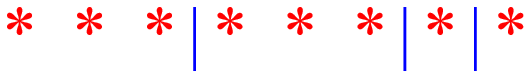This corresponds to the arrangement: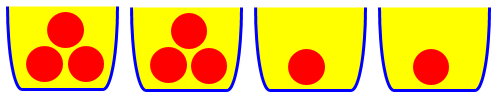This method leads to the general formula (for $$b$$ balls in $$u$$ urns, again, where we put $$u-1$$ bars into $$b-1$$ gaps) $${{b-1}\choose{b-u}}\text{ or }{{b-1}\choose{u-1}}.$$

Again, we can check our work by either actually listing all possibilities, or by imagining doing so and using some shortcuts:

Finally, I want to suggest that 35 isn't too many to count. You could just literally make a list. I think this is useful - in fact all combinatorial formulas begin with counting. As you invent symbols for the objects you want to count, you just naturally begin to categorize and group them. Then you see patterns in how many symbols there are in each group, and you begin to move toward a general formula.

In this case, you might say:  8 apples could be distributed 5-1-1-1, or 1-5-1-1, or 1-1-5-1, or 1-1-1-5. But all these are the same, except for ordering. It would be a good shortcut to just write 5-1-1-1 and note that there are 4 different positions to put the 5, so we count 4 partitions of this type.

Similarly, 4-2-1-1 has 12 orderings, C(4,2), since there are 4 possibilities for which child gets the 4 times 3 possibilities for which other child gets the 2.

Continuing: 3-3-1-1 has 6 orderings, and 3-2-2-1 has 12 orderings, while 2-2-2-2 has just 1.

5111         4
4211        12
3311         6
3221        12
2222         1
------
35

## A different way

Something neither Doctor Anthony or Doctor Mitteldorf did is to show an alternative calculation. Think about this: In order to ensure that each child gets at least one apple, we could just give one to each, and then use the method we used previously! That is, we use up 4 of the apples, and then distribute the remaining 4 apples to the 4 children, allowing some to get none. Our previous formula results in $$\displaystyle{{4+4-1}\choose{4}} = {7\choose 4} = 35$$ — the same answer!

By the same thinking, we can produce a new formula for the case where at least one ball must be in each urn: $${{(b-u)+u-1}\choose{b}} = {{b-1}\choose{b-u}}\text{ or }{{b-1}\choose{u-1}},$$ as before.

## Rock-Paper-Scissors

Let’s look at one more problem using this technique, from 2014:

From Rock-Paper-Scissors to Stars and Bars

Okay, I know the answer to this problem, but I don't know why the combination formula cannot be used to solve it. Please explain that to me.

Three friends are playing a game in which each person simultaneously displays one of three hand signs: a clenched fist, an open palm, or two extended fingers. How many different combinations of the signs are possible?

If I use 9 for the number of possible ways (3 friends * 3 signs) and 3 for the number of signs, and plug them into the combination formula ... well, you see 9!/3!(6!) doesn't work. The permutation formula doesn't work either. WHY NOT?

Writing it all out does work:

Let's call clenched fist C; open palm O; and two fingers F. The possibilities are:

CCC   OOO   FFF
COO   OCC   FCC
CFF   OFF   FOO
COF

That's 10 different combinations.
WHY can't I use C(n,r) = n!/r!(n - r)!?

Because order is being ignored (it doesn’t matter who makes what sign), this isn’t a permutation problem; but it also isn’t a combination problem in the usual sense, because repetitions are allowed.

You may notice that I previously referred to an answer to the same problem from 2001, which I evidently didn’t know about when I wrote this answer; but that gave me a chance to give a deeper explanation. I might have use the notation RPF (Rock, Paper, Scissors), but those terms weren’t used in the question, and I chose to stick with KC’s notation.

I have a method you might like. It's commonly called "stars and bars."

You want to find the number of ways to fill three blanks with C, O, or F, allowing repetition, but ignoring order. So we can assume that every such combination is written in that order, C, O, F.

This comment relates to a standard way to list combinations. By always writing the elements in the same order, we are actually ignoring order — in effect, representing all possible orderings of a given combination by one standard ordering.

This is equivalent to finding three numbers whose sum is 3, where each number can be 0, 1, 2, or 3. For example, the combination OFF is

0C + 1O + 2F
0 +  1 +  2 = 3.

Here we have a second model of the problem, as a mere sum. The order implies meaning; the first number in the sum is the number of closed fists, and so on. This is reminiscent of the way in which matrices are used to represent a system of equations, the first number being the coefficient of x, the second of y, and so on. We are abstracting away all direct reference to meaning, turning a multiset into a mere list of numbers.

We can picture this by making 3 bins to hold the number of C, O, and F respectively, and putting a total of 3 "stars" into them:

C   O   F
|   | * |* *|

This in turn is equivalent to starting with 3 stars, and placing four "bars" among them (making sure there is at least one bar on each end):

| | * | *   * |

Since the first and last bars are always in the same places, we can ignore them, and just place two bars among the stars:

| * | * *


We have made a series of models, each time re-imagining an existing representation as another that we might be able to count more easily. Multiple representations are a key idea for learning math well.

Now, if we couldn't have zero, this would translate directly to choosing 2 of the 4 places where bars can be put.

But unfortunately, we still don't have a combination problem, because we can put two or more bars in the same place, like so:

*||* *

We saw this approach (filling spaces) in the last problem, where zero wasn’t allowed. But it is allowed here (no one has to make any particular sign). So we have to count arrangements in a way that allows any arrangement of the two bars and three stars — which is exactly what the basic combination formula does:

So let's turn the problem inside-out yet again! We have three stars and two bars, AND want to find the number of ways to arrange ALL of them in order. That is, there are five spaces, and we want to put stars in three of them and bars in the other two.

Now we see that the problem is equivalent to choosing two of five places to put bars, which (finally!) is a simple combination problem:

C(5, 2) = 5!/(2!3!) = 10

There's your answer!

And the combination formula is usable, just not in the simple way KC envisioned. It’s the formula from our first example, $${{b+u-1}\choose{u-1}} = {{3+3-1}\choose{3-1}} = {5\choose 2} = 10,$$ with 3 “balls” (indistinguishable hands) in 3 “urns” (distinguishable signs).

And, just for fun, let's list the ten ways to arrange the stars and bars, and which answers they relate to, in terms of numbers or of rock-paper-scissors (that is, closed fist, open hand, two fingers):

||***     0 + 0 + 3     FFF
|*|**     0 + 1 + 2     OFF
|**|*     0 + 2 + 1     OOF
|***|     0 + 3 + 0     OOO
*||**     1 + 0 + 2     CFF
*|*|*     1 + 1 + 1     COF
*|**|     1 + 2 + 0     COO
**||*     2 + 0 + 1     CCF
**|*|     2 + 1 + 0     CCO
***||     3 + 0 + 0     CCC

That was fun! I actually didn't know what form the answer would take when I started writing, but just knew that stars and bars would almost certainly work.

This is the same list KC had, but in an orderly form.

### 3 thoughts on “Stars and Bars: Counting Ways to Distribute Items”

1.What if you take the apples problem an make it even more twisted. Assume that you have 8 identical apples and 3 children. Each child is supposed to receive at least one apple, but no child is supposed to get more than 3 apples in total. How would you solve this problem?

1.My first impression when I read your question was that, in general, this type of problem is much more complicated than what we discussed in this post. When you add restrictions like a maximum for each, you make the counting harder. I suspect that the best method for such problems would be generating functions (something I never learned). It’s not hard to “twist” a combinatorics problem and make it impossible to do without just counting everything one by one.

But my second thought is that a new problem has to be looked at on its own; any problem may have its own special trick. That is true here, because of the specific numbers you used.

Did you notice that if each child got the maximum, you would use only 9 apples, 1 more than the number you have? This makes it easy. Rather then give apples to each of them, give each of them 3 IOU’s for apples, and then you just have to count the number of ways to take an IOU away from one child, after which you would redeem them! How many ways can you take away one IOU? That’s easy. But if you change the numbers (say, allowing a higher individual maximum, or more total apples), things will quickly get more complicated.

This site uses Akismet to reduce spam. Learn how your comment data is processed.